Why two popular textbook equations are actually the same

Now that we have that out of the way, let’s proceed. Today, we’ll do a little exercise, and show that two popular calculation approaches are indeed fully equal.

Without further ado, let’s dive in and check out the two methods.

The first approach is commonly found in textbooks. It consists of first defining the so-called tangential stress or magnetic shear stress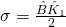,

where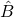and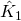are the peak values of the (first spatial harmonic of) airgap flux density and linear current density.

Then, the torque is obtained by first multiplying the stress by the airgap surface area of the rotor (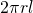) to obtain a force of a kind. The torque is then the force times the moment arm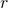, resulting in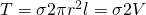.

Meaning, for a given tangential stress, the torque is proportional to twice the volume enclosed by the airgap. As you can guess, this makes the stress approach very useful for quickly estimating the motor size needed for reaching a certain torque.

### K-what??

However, in my experience, the linear current densities vary all over the place once you consider anything other than the rated torque of a standard industrial motor. In other words, don’t trust the textbook values too far.

Luckily, the linear current density is very easy to calculate, being equal to the total slot-bound current (peak Ampere-turns) divided by the slot pitch (airgap circumference divided by the number of slots).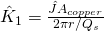.

If this sounds too simplistic for you, worry not. It does. Sound too simple, I mean. After all, one should consider fundamental harmonic amplitudes when computing to torque. However, for a balanced three-phase winding, the first spatial harmonic of the linear current density distribution IS equal to the aforedescribed expression, a fact easily-but tediously shown by applying some math.

#### Mini-rant

Furthermore, if the expression above seems unfamiliar to you, it may be thanks to a conscious choice of mine. Indeed, many authors and books express the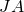term with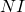instead.

I strongly dislike the practice. To begin with, the phase current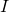and the number of turns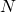can both be varied inversely proportional to each other, and still end up with exactly the same performance. (As a first-order approximation, skin-effect losses etc. will of course change.)

By contrast, using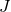and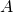directly ties the linear current density to the machine size, geometry, and cooling. Everybody understands these as hard constraints, something not easily bent. In other words, it’s hard for even a beginner to fool themselves with “let’s double the number of turns to get twice to torque!!“, or anything similar. Not that I know anything about that.

In any case, the number of turns can later be selected more or less freely to match the supply voltage, after which the phase current is obtained from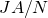, essentially.

## Lorentz force

The second approach today is based on the Lorentz force formula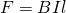,

for a conductor of lengthcarrying a currentin flux density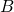.

In the torque computation context, the formula takes the form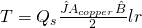,

where the division by 2 appears since we are using peak values now, mind you.

Compared to the tangential stress method, the Lorentz force approach is much more commonly found online and in introductory materials.

Sidenote: Sadly, THEmost common approach is still something like “if the motor voltage is 400 V and the current is 200 A…” Yeah, let’s make the motor thumb-sized, too, while we’re at it. Computing the torque from current and voltage is all nice and cool if you already have the motor, but useless for designing one. The product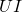has to come from something, and that something is – guess what – proportional to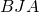, again. Those three are The Quantities you just have to respect.

## Equivalence

Now, let’s see how these two approaches are indeed fully equivalent. First, let’s collect the formulas for the tangential stress, torque, and the definition of electric loading:

By combining, we get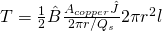.

This can immediately be simplified to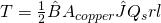,

which, after re-ordering, is exactly the same as the Lorentz force approach.

Woo, that was simple!

### Why the approaches are equivalent

Now, let’s tackle the harder question: Why does this happen?

After all, we are speaking about approximate calculation methods here – not an exact solution to the Maxwell’s equations. Thus, one could expect two different methods to give something in the same neighbourhood, not exactly the same.

Well, it turns out the methods aren’t different at all, after all.

You remember, the tangential stress is computed from the electric loading or linear current density. Or surface current density, if you’re thinking in correct terms.

Like you should.

Digressing again.

Right, the surface current density, like its name suggests, is a virtual current flowing in a very thin layer on the airgap surface. In the tangential stress approach, it is assumed to vary sinusoidally in space and time.

However, the Lorentz force approach is in fact also based on a surface current of kind. The only difference is that the current is no longer assumed spread along the entire airgap surface. Instead, it is concentrated on infinitely small points (lines in 3D), each corresponding to a slot. Think delta functionals, if you know some maths.

### Of motors and harmonics

Now, this is where it gets interesting. Turns out the sinusoidal current density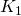used in the shear stress approach is the fundamental spatial harmonic – the first Fourier series term with respect to the angular position – of the point-wise current distribution (let’s call it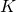) of the Lorentz approach. This is relatively easy to show by some calculations – try it!

As we remember, the torque is them calculated by integrating the product of the airgap flux density and linear current density over the airgap surface (multiplied by radius).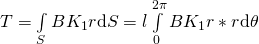.

Also remember that the flux density was assumed sinusoidal with respect to the angular coordinate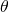.

Now, we want to calculate the integral of the product of two functions,and, around the airgap circumference. One if these functions,, is sinusoidal, and the integral length (airgap circumference) is an integer multiple (pole-pair number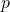) of its wavelength. Now, thanks to old fella’ Fourier we know that it doesn’t matter at all if we usedirectly as the other function, or its first harmonic. The integrals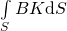and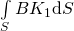will be exactly the same!

## Conclusion

Two common approaches – magnetic shear stress and Lorentz force – should predict exactly the same torque for an electric motor. Not approximately same, mind you, but exactly.

This happens because they are not really separate methods at all. Rather, the former is a special case of the latter.

Check out EMDtool - Electric Motor Design toolbox for Matlab.

Need help with electric motor design or design software? Let's get in touch - satisfaction guaranteed!Shear Stress versus Lorentz Force